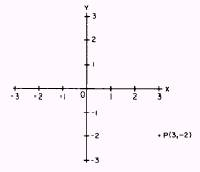Custom SearchPre-Calculus and Intro to ProbabilityDistance Between Two Points### CHAPTER 1 STRAIGHT LINES

LEARNING OBJECTIVES

Upon completion of this chapter, you should be able to do the following:

1. Calculate the distance between two points.

2. Locate a point by dividing a line segment.

3. Define the inclination of a line and determine the line's slope.

4. Solve for the slopes of parallel and perpendicular lines.

5. Compute the angle between two lines.

6. Determine the equation of a straight line using the point-slope form, the slope-intercept form, and the normal form.

7. Determine the equations of parallel and perpendicular lines.

8. Calculate the distance from a point to a line.

INTRODUCTION

The study of straight lines provides an excellent introduction to analytic geometry. As its name implies, this branch of mathematics is concerned with geometrical relationships. However, in contrast to plane and solid geometry, the study of these relationships in analytic geometry is accomplished by algebraic analysis.

The invention of the rectangular coordinate system made algebraic analysis of geometrical relationships possible. Rene Descartes, a French mathematician, is credited with this invenŁtion; the coordinate system is often designated as the Cartesian coordinate system in his honor.

You should recall our study of the rectangular coordinate system in Mathematics, Volume l, NAVEDTRA 10069-D1, in which we reviewed the following definitions and terms:

1. The values of x along, or parallel to, the X axis are abscissas. They are positive if measured to the right of the origin; they are negative if measured to the left of the origin. (See fig. 1-1.)

2. The values of y along, or parallel to, the Y axis are ordinates. They are positive if measured above the origin; they are negative if measured below the origin.

3. The abscissa and ordinate of a point are its coordinates.

Any point on the coordinate system is designated by naming its abscissa and ordinate. For example, the abscissa of point P (fig. 1-1) is 3 and the ordinate is - 2. Therefore, the symbolic notation for P is

P(3, -2)Figure 1-1.-Rectangular coordinate system.

In using this symbol to designate a point, the abscissa is always written first, followed by a comma. The ordinate is written last. The general form of the symbol is

P(x,y)